Courses

# Bending & Shear Stress in Beams - 2 Civil Engineering (CE) Notes | EduRev

## Civil Engineering (CE) : Bending & Shear Stress in Beams - 2 Civil Engineering (CE) Notes | EduRev

The document Bending & Shear Stress in Beams - 2 Civil Engineering (CE) Notes | EduRev is a part of the Civil Engineering (CE) Course Solid Mechanics.
All you need of Civil Engineering (CE) at this link: Civil Engineering (CE)

Simple Bending Theory

When a beam having an arbitrary cross section is subjected to transverse loads the beam will bend. In addition to bending the other effects such as twisting and buckling may occur, and to investigate a problem that includes all the combined effects of bending, twisting and buckling could become a complicated one. Thus we are interested to investigate the bending effects alone, in order to do so, we have to put certain constraints on the geometry of the beam and the manner of loading.

1. Assumptions
The constraints put on the geometry would form the assumptions:

• Beam is initially straight, and has a constant cross-section.
• Beam is made of homogeneous material and the beam has a longitudinal plane of symmetry.
• Resultant of the applied loads lies in the plane of symmetry.
• The geometry of the overall member is such that not the bending,it is the buckling that is the primary cause of failure.
• Elastic limit is nowhere exceeded and ‘E' is same in tension and compression.
• Plane cross - sections remains plane before and after bending.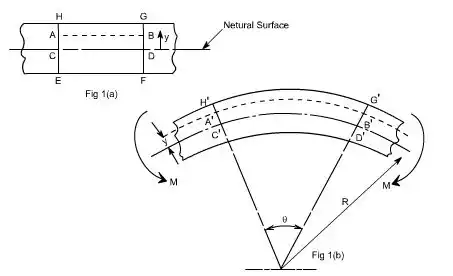Let us consider a beam initially unstressed as shown in fig 1(a). Now the beam is subjected to a constant bending moment (i.e. ‘Zero Shearing Force') along its length as would be obtained by applying equal couples at each end. The beam will bend to the radius R as shown in Fig 1(b)
As a result of this bending, the top fibers of the beam will be subjected to tension and the bottom to compression it is reasonable to suppose, therefore, that some where between the two there are points at which the stress is zero. The locus of all such points is known as neutral axis. The radius of curvature R is then measured to this axis. For symmetrical sections the N. A. is the axis of symmetry but what ever the section N. A. will always pass through the centre of the area or centroid.
The above restrictions have been taken so as to eliminate the possibility of 'twisting' of the beam.

The concept of pure bending

As we are aware of the fact internal reactions developed on any cross-section of a beam may consists of a resultant normal force, a resultant shear force and a resultant couple. In order to ensure that the bending effects alone are investigated, we shall put a constraint on the loading such that the resultant normal and the resultant shear forces are zero on any cross-section perpendicular to the longitudinal axis of the member,
That means F = 0,
or M = constant.
Thus, the zero shear force means that the bending moment is constant or the bending is same at every cross-section of the beam. Such a situation may be visualized or envisaged when the beam or some portion of the beam, as been loaded only by pure couples at its ends. It must be recalled that the couples are assumed to be loaded in the plane of symmetry.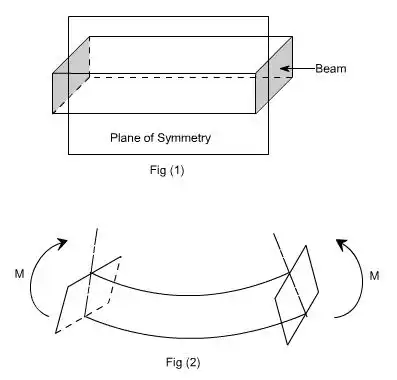When a member is loaded in such a fashion it is said to be in pure bending. The examples of pure bending have been indicated in EX1 and EX2 as shown below: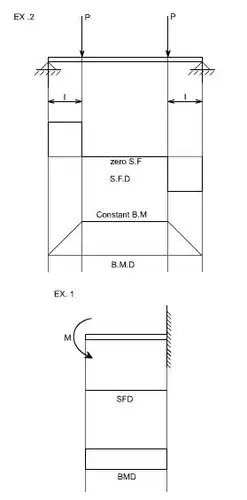When a beam is subjected to pure bending are loaded by the couples at the ends, certain cross-section gets deformed and we shall have to make out the conclusion that,
(a) Plane sections originally perpendicular to longitudinal axis of the beam remain plane and perpendicular to the longitudinal axis even after bending, i.e. the cross-section A'E', B'F' (refer Fig 1(a)) do not get warped or curved.
(b) In the deformed section, the planes of this cross-section have a common intersection i.e. any time originally parallel to the longitudinal axis of the beam becomes an arc of circle.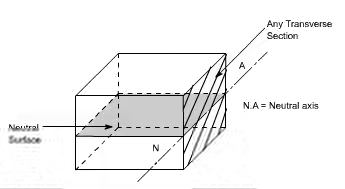We know that when a beam is under bending the fibres at the top will be lengthened while at the bottom will be shortened provided the bending moment M acts at the ends. In between these there are some fibres which remain unchanged in length that is they are not strained, that is they do not carry any stress. The plane containing such fibres is called neutral surface.
The line of intersection between the neutral surface and the transverse exploratory section is called the neutral axis neutral axis (N A) .
2. Bending Stresses in Beams
M / I = E / R = σ / y
This equation is known as the Bending Theory Equation.
The above proof has involved the assumption of pure bending without any shear force being present. Therefore this termed as the pure bending equation. This equation gives distribution of stresses which are normal to cross-section i.e. in x-direction.
Useful formulas for Ixx, Iyy, Zxx & Zyy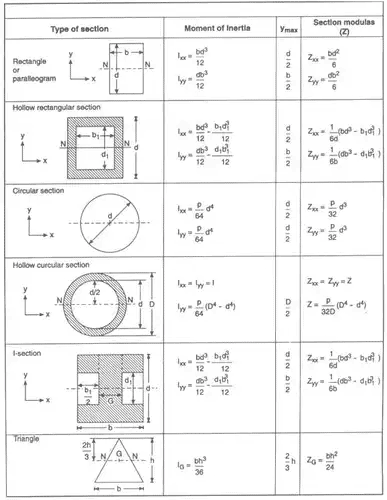Offer running on EduRev: Apply code STAYHOME200 to get INR 200 off on our premium plan EduRev Infinity!

## Solid Mechanics

31 videos|27 docs|29 tests

,

,

,

,

,

,

,

,

,

,

,

,

,

,

,

,

,

,

,

,

,

;